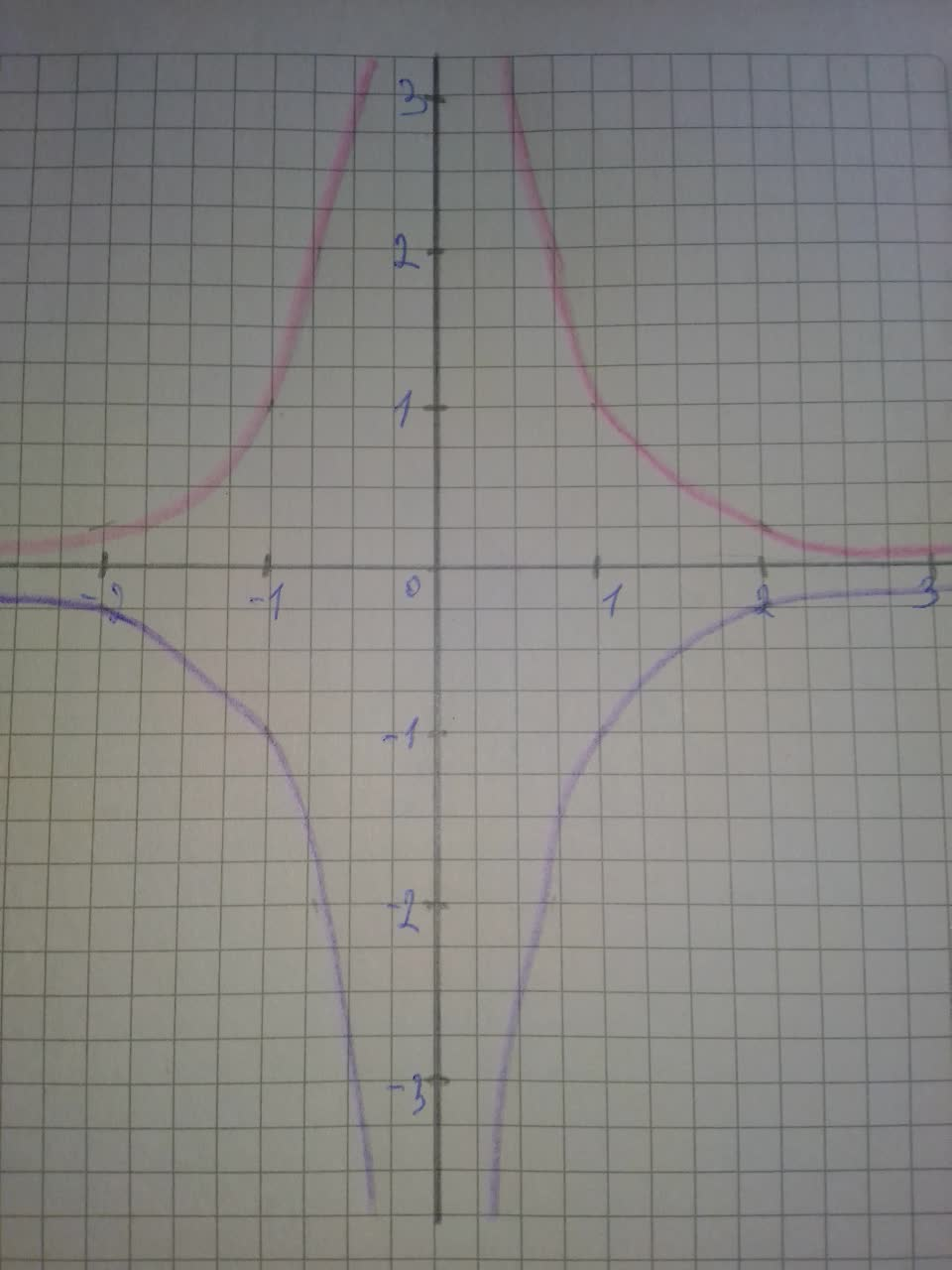# Sketch a graph of the function. Use transformations of functionsCoormaBak9 2021-08-14 Answered
Sketch a graph of the function. Use transformations of functions whenever possible. $f\left(x\right)=-\frac{1}{{x}^{2}}$
You can still ask an expert for help

• Questions are typically answered in as fast as 30 minutes

Solve your problem for the price of one coffee

• Math expert for every subject
• Pay only if we can solve itfirmablogF
Step 1
First graph function $y=\frac{1}{{x}^{2}}$
Then, to obtain graph of function $f\left(x\right)=-\frac{1}{{x}^{2}}$, do following transformations:
-reflect with respect to x-axis
On graph:
Red -$y=\frac{1}{{x}^{2}}$
Blue - $f\left(x\right)=-\frac{1}{{x}^{2}}$
Step 2